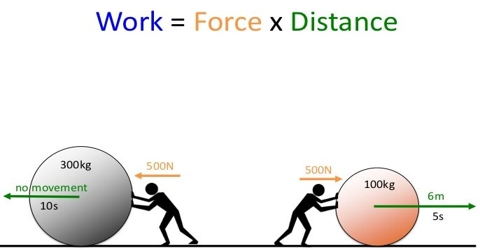Physics

# Concept of WorkConcept of Work

If there is displacement of a body due to application of force only then work is done. For example, a book is allowed to fall from the table to ground. A person reached to the top of a hill step by step by carrying a load on his head. By these two examples, we explain work. In the first case, displacement has been done along the direction of gravitational force and in the second case; displacement has been done against the gravitational force. So, work has been done in both the cases. A person is standing carrying load on his shoulder became tired but no work is done in this case as there is no displacement. From this discussion it is understood that- if there is displacement due to application of force only then work will be done. But if there is no displacement even if force is applied, no work will be done.

Unit of work: Unit of work is Newton-metre (N-m). It is called Joule (J). That means, 1 Joule = 1 Newton x 1 metre

In our daily life we see many examples of work around us. Boys are playing football and rickshaw pullers is pulling rickshaw, vendors are selling different products, the cultivator is ploughing in the field etc.

So work can be defined in the following way:

If force is applied on a body and if there is a displacement of the body, then product of the force and the component of displacement along the direction of force is called work.